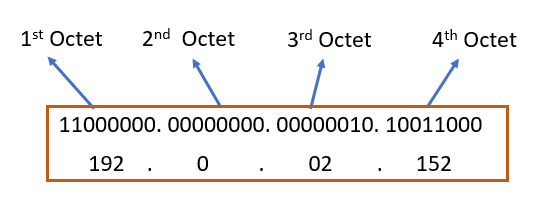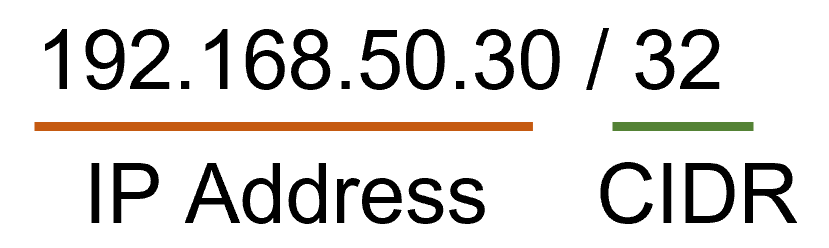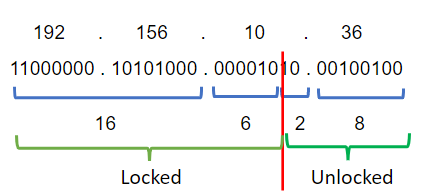# What is the Range of IP Address

Home /

#### Related Tutorials

Devices on a network are uniquely identified by their IP addresses, which are numerical identifiers. The Internet Protocol (IP) version being utilized determines the IP address range. IP addresses are 32-bit integers with four portions, each ranging from 0 to 255, in IPv4, the most widely used version. There are hence around 4.3 billion distinct IP addresses in all. Nevertheless, IPv4 is gradually being phased out in favor of IPv6, which utilizes 128-bit addresses and can handle a far wider variety of unique addresses, due to the restricted amount of addresses that are now accessible. The over 340 undecillion distinct IP addresses that IPv6 supports ensure that there will always be enough addresses to support networks and devices in the future.

The Internet Protocol version 4 (IPv4) is the fourth version of the Internet Protocol (IP) and the first extensively implemented version of the protocol. IP addresses (version 4) are 32-bit integers that may be written in hexadecimal format. The most common format is x.x.x.x, which is also known as dotted quad or dotted decimal. Each x is termed an octet since it is an 8-bit value. Therefore, in decimal notation, the range of x can be 0 to 255. For example, 192.0.2.152 is a valid IPv4 address.Fig 1: Structure of IPv4

Each router has a range of IP addresses that it may use. For instance, suppose a router’s IP address range is 192.168.50.0 to 192.168.50.200. As an outcome, there are a total of 201 IP addresses. However, the first IP (network IP) and the last IP (broadcast IP) are reserved. So,, the total number of IP addresses accessible is 199 for this router.

## Drawbacks of class-based IP addressing

All accessible IP addresses in the classful routing system are classified into distinct classes. As a result, an organization must utilize an IP address of class A, B, or C. Different types of classes, their range, and the number of host identifiers are presented in the table below.

When an organization needed more than 254 host Identifiers, this routing system would frequently fail.  Because the organization would now be classified as class B rather than class C. This indicates that the organization would utilize a class B license while having fewer than 65,535 hosts. That is, if an organization requires 535 IP addresses, it falls into class B. In this manner, the company will waste the remaining 65000 IP addresses, reducing the availability of IPv4 addresses needlessly. The Internet Engineering Task Force (IETF) created CIDR in 1993 to address this issue.

## Classless Inter-Domain Routing (CIDR)

CIDR, commonly known as supernetting, is a technique of allocating Internet Protocol (IP) addresses that enhances address distribution efficiency. The former system, which was based on Class A, Class B, and Class C networks, has been replaced by the CIDR scheme. This approach also aided in extending the life of IPv4 and reducing the size of routing tables.

Prefix and suffix are two sets of integers that make up CIDR IP addresses. The prefix denotes a network address (192.255.255.255), while the suffix denotes the number of bits in the complete address (12). The slash(/) symbol separates the prefix and suffix. A CIDR IP address might look something like this when put together:Fig 2: Structure of classless routing system

The CIDR system is used to define IP ranges. Its maximum value is 32, which corresponds to the number of bits in an IP address. In Fig 2, the value of CIDR is 32. This indicates that there is only one IP address possible in this scenario. But when the value of CIDR is other than 32, then it refers to a range of IPs. Now let’s see a few other examples.

There are 32 bits in each IP address x.x.x.x. We may deduce from the table that CIDR = 24 indicates that the first 24 bits are locked and the other 8 bits can freely choose any number from 0 to 255. So, when CIDR = 16, locked bits would be 16, and so on. So, the total number of IP addresses can be found in the equation given below.

no of IP address =232 – CIDR – 2

So, if CIDR = 16, the total IP address would be 232-16 – 2 = 65534.

So far we have analyzed the CIDR which has a value of 2th power. Therefore, the question remains what would be the case if the value of CIDR is not 2th power such as 15 or 21? Let’s dig into one of these examples.

Example 1:  For an IP 192.168.10.36 / 22, find out the no of the IP address possible and the IP address range.

Solution:

No of the IP address  = 232-22 -2= 1022.

In the problem here, CIDR = 22. Therefore, we may safely conclude that the first 16 bits of the 2 octets are locked. From here, we must convert the decimal value of IP to a binary number in order to approach the problem.Fig: Graphical presentation of the problem.

As a result, the first 22 bits are locked, leaving the remaining 10 bits free to accept any value they choose. The number of unlocked bits in the third octet is now two. In conclusion, the minimum value of these 2 bits is 00 and the greatest value is 11.

Therefore, starting IP: 11000000 . 10101000 . 00001000 . 00000000

In decimal,                       192       .     156       .        8        .       0

and, ending  IP:          11000000 . 10101000 . 00001011 . 11111111

In decimal,                        192      .     156       .        11      .     255

Hence, the IP address range is 192.156.8.0 to 192.156.11.255. Because the first and final IP addresses are always reserved, the usable IP range is 192.156.8.1 – 192.156.11.254.

Example 2:   For an IP 10.10.0.0 / 16, find out the no of the usable IP address and IP address range.

Solution:

No of the IP address  = 232-16 -2= 65534 .

So, here, CIDR = 16 which means the initial 16 bits are locked.

Hence, the IP range: 10.10.0.0 – 10.10.255.255

Usable IP range: 10.10.0.1 – 10.10.255.254

For the readers’ convenience, some practice problems are provided below.

• 10.156.58.15 / 15
• 168.192.0.0 / 25
• 172.29.0.0 / 28